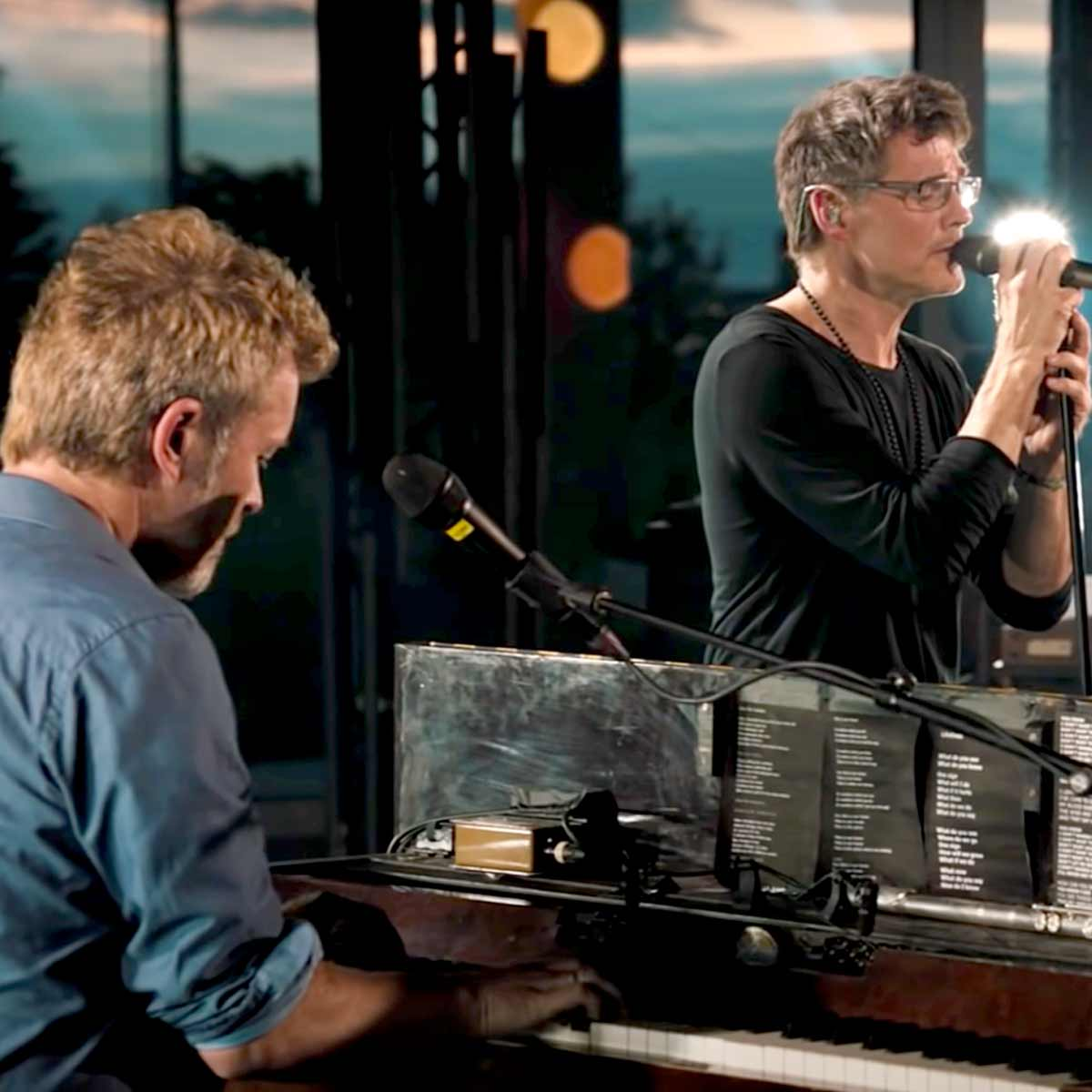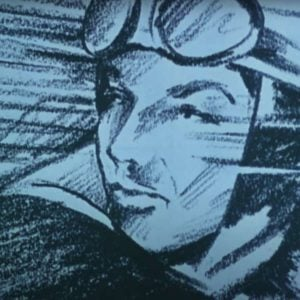# A-ha

2 Music Sheets

• ###### Genres:

A-ha is a Norwegian synth-pop band formed in Oslo in 1982. Founded by Paul Waaktaar-Savoy (guitars), Magne Furuholmen (keyboards, guitars), and Morten Harket (vocals), the band rose to fame during the mid-1980s. A-ha achieved their biggest success with their debut album Hunting High and Low in 1985. That album peaked at number one in their native country Norway, number 2 in the UK, and number 15 on the US Billboard album chart; yielded two international number-one singles: "Take On Me" and "The Sun Always Shines on T.V."; and earned the band a Grammy Award nomination as Best New Artist. In the UK, Hunting High and Low continued its chart success into the following year, becoming one of the best-selling albums of 1986.

Credit: Wikipedia

## Artist's Music Sheets

•[9z] c b y 9 y [9c] y 9 y [9x] y l 9 y 9 y 9 y d [9jz] y d 9 y d [9jz] [jz] y d 9 y d [9jz] y x 9 [yl] 9 y 9 y [9b] b [yc] z [9z] y v w v o v n [wn] [om] [8m] m [tm] v [8c] t b q b i b [0v] v [ub] v [9b] b [yc] z [9z] y v w v o v n [wn] [om] [8m] m [tm] v [8c] t b q b i b [0v] v [ub] v [9b] b [yc] z [9z] y v w v o v n [wn] [om] [9m] m [ym] v [9c] y b w b o b v [wv] [ob] v [9g] y g [9f] [yd] w o w o [8f] f t f [8s] t q j i j [0j] [uh] [9g] y g g [9f] y d w o w o d [8f] [tg] f [8d] t s q d i f [0d] [us] 9 [yg] g 9 [yg] g w o w o e [ps] s s [es] [ps] s [qs] a i [qa] p i [8us] t 8 t [7dhk] r 7 r [6fjl] e 6 e [isgh] q [isgj] q [isgh] [8fhl] t 8 t [7kzv] r 7 r [6lxb] e 6 e [isgh] q [isgj] q [isgh] [8hlx] 8 t 8 [7zvn] 7 7 r 7 [6xbm] 6 6 e 6 [4q] n m n [bm] 8 [tm] 8 t [7dhk] r 7 r [qsgj] i q i [wdhk] o w h o [9g] y g [9f] [yd] w o w o d [8f] [tg] f [8d] [ts] q j [ij] [0j] [uh] [9g] y g [9f] y d w o w o [8f] [tg] f [8d] [ts] s q d [if] [0d] [us] [9g] [yg] g [9g] [yg] w o w o e [ps] s s [es] [ps] s [qs] [ia] a q p i [8us] t 8 t [7dhk] r 7 r [6fjl] e 6 e [isgh] q [isgj] q [isgh] [8fhl] t 8 t [7kzv] r 7 r [6lxb] e 6 e [qsgh] 4 [qsgj] 4 [qsgh] [8hlx] 8 8 t 8 [7zvn] 7 r 7 [6xbm] 6 6 e 6 [4q] n m m n [bm] 8 [tm] 8 t [7dhk] r 7 r [4sgj] q 4 q [5dhk] w 5 w [0xvn] u 0 u [0v] u 0 u [^Jgz] E ^ E ^ E ^ E [0xvn] u 0 u [0vn] u 0 u [^Jgz] E ^ E ^ E [lx] ^ E [9lx] [zc] y 9 y 9 y [jl] 9 y [wjl] [kz] o w o [wk] o w o b b c z z v v v n n m b b c z z v v v n n m [9b] b [yc] z [9z] y v w v o v n [wn] [om] [8m] m t v [8c] t b q b i b [0v] v [ub] v [9b] b [yc] z [9z] y v w v o v n [wn] [om] [8m] m [tm] v [8c] t b q b i b [0v] v [ub] v [9b] b [yc] z [9z] y v w v o v n [wn] [om] [9m] m [ym] v [9c] y b w b o b [wv] o [9g] y h [9j] [yh] w o [wg] o [8f] [tg] f [8s] t q j i j [0j] [uh] [9g] y g [9f] [yd] [wd] o w o s [8f] [tg] f [8d] t s q d i f [0d] [us] 9 y g [9g] [yg] g w o w o e [ps] s s [es] [ps] s q a [ia] a [qp] i [8us] t 8 t [7dhk] r 7 r [6fjl] e 6 e [isgh] q [isgj] q [isgh] [8fhl] t 8 t [7kzv] r 7 r [6lxb] e 6 e [isgh] q [isgj] q [isgh] [8hlx] 8 8 t 8 [7zvn] 7 7 r 7 [6xbm] 6 6 e 6 [4q] [cb] [vn] 8 [tm] 8 t [7dhk] r 7 r [6fjl] e 6 e [qsgh] 4 [qsgj] 4 [qsgh] [8fhl] t 8 t [7kzv] r 7 r [6lxb] e 6 e [qsgh] 4 [qsgj] 4 [qsgh] [8hlx] t 8 t [7zvn] r 7 r [6xbm] e 6 e 4 q [4xv] [xv] q 8 [tm] 8 t [7kzv] r 7 r [6lxb] e 6 e [isgh] q [isgj] q [isgh] [8tsf]

Level: 6
Length: 03:28
Intermediate

#### A-ha

•GGda a f f fHHjkjjjf d G G GffGf GGda a f f fHHjkjjjf d G G GffGf GGda a f f fHHjkjjjf d G G GffGf d|d Sa| SSSS p|G GGf d|d Sa| aS dS a p a Sa p d|dddd|| ppppp p OO I e||u||I|ffG f e||u|I | fffG f T||O||p|| uu f
Level: 3
Length: 00:50
Easy